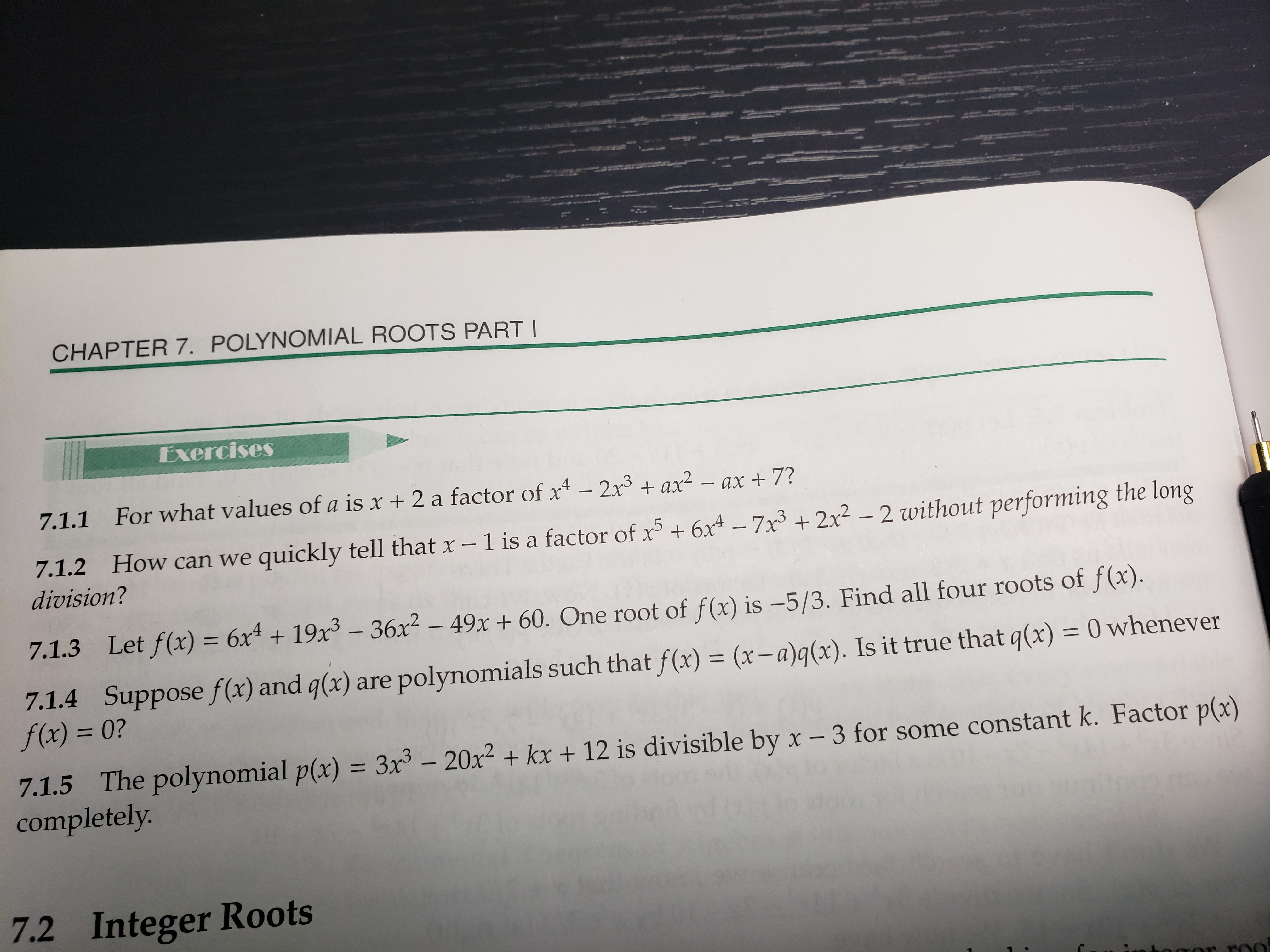# CHAPTER 7. POLYNOMIAL ROOTS PARTI7.1.1 For what values of a is x + 2 a factor of x4 2x3+ax2 - ax +7?ow can we quickly tell that x - 1 is a facto of xx4 - 7x3 +2x2 - 2 without performing the longdivision?7.1.3Let f(x) = 6x4 + 19x3-36x2-49x + 60. One root of f(x) is-5/3. Find all four roots of f(x).7.1.4 Suppose fCx) and gx) are polynomials such that f(e) (r-)(). Is it true that q(x)f(x) = 0?0 whenever7.1.5 The polynomial p/x) 3x-20+ k + 12 is divisible by x -3 for some constant k. Factor p:)completely7.2 Integer Roots

Question
11 views

Question 7.1.3help_outlineImage TranscriptioncloseCHAPTER 7. POLYNOMIAL ROOTS PARTI 7.1.1 For what values of a is x + 2 a factor of x4 2x3+ax2 - ax +7? ow can we quickly tell that x - 1 is a facto of xx4 - 7x3 +2x2 - 2 without performing the long division? 7.1.3 Let f(x) = 6x4 + 19x3-36x2-49x + 60. One root of f(x) is-5/3. Find all four roots of f(x). 7.1.4 Suppose fCx) and gx) are polynomials such that f(e) (r-)(). Is it true that q(x) f(x) = 0? 0 whenever 7.1.5 The polynomial p/x) 3x-20+ k + 12 is divisible by x -3 for some constant k. Factor p:) completely 7.2 Integer Roots fullscreen
check_circle

Step 1

The first factor is already given. Use synthetic division in order to find the partially factored f(x).

Step 2

For more real roots, try placing more numbers in the cubic part g(x) of the partially factored f(x). Place x=1 and see

Step 3

Use synthetic division in order to fi...

### Want to see the full answer?

See Solution

#### Want to see this answer and more?

Solutions are written by subject experts who are available 24/7. Questions are typically answered within 1 hour.*

See Solution
*Response times may vary by subject and question.
Tagged in
MathAlgebra

### Polynomials